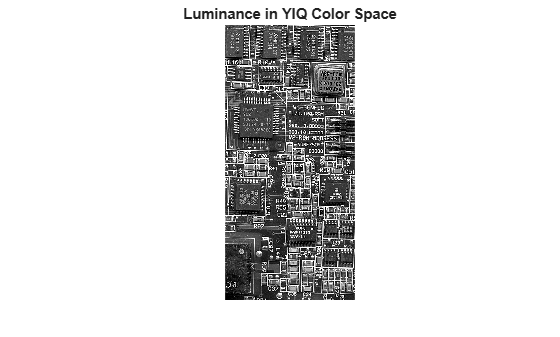Documentation

# rgb2ntsc

Convert RGB color values to NTSC color space

## Syntax

``YIQ = rgb2ntsc(RGB)``
``yiqmap = rgb2ntsc(rgbmap)``

## Description

example

````YIQ = rgb2ntsc(RGB)` converts the red, green, and blue values of an RGB image to luminance (Y) and chrominance (I and Q) values of an NTSC image.```
````yiqmap = rgb2ntsc(rgbmap)` converts an RGB colormap to an HSV colormap.```

## Examples

collapse all

This example shows how to convert an image from RGB to NTSC color space.

Read an RGB image into the workspace.

`RGB = imread('board.tif');`

Convert the image to YIQ color space.

`YIQ = rgb2ntsc(RGB);`

Display the NTSC luminance value, represented by the first color channel in the YIQ image.

```imshow(YIQ(:,:,1)); title('Luminance in YIQ Color Space');```## Input Arguments

collapse all

RGB image to convert, specified as an m-by-n-by-3 numeric array with values in the range [0, 1]. The third dimension of `RGB` defines the red, green, and blue intensity of each pixel, respectively.

Data Types: `double`

RGB colormap, specified as a c-by-3 numeric matrix with values in the range [0, 1]. Each row of `rgbmap` is a three-element RGB triplet that specifies the red, green, and blue components of a single color of the colormap.

Data Types: `double`

## Output Arguments

collapse all

NTSC image, returned as an m-by-n-by-3 numeric array with values in the range [0, 1]. The third dimension of `YIQ` defines the luminance (Y) and chrominance (I and Q) for each pixel, respectively

Data Types: `double`

NTSC colormap corresponding to the c colors in `rgbmap`, returned as a `c`-by-3 numeric matrix with values in the range [0, 1]. Each row of `yiqmap` is a three-element YIQ triplet that specifies the luminance (Y) and chrominance (I and Q) components of a single color of the colormap.

Data Types: `double`

## Algorithms

In the NTSC color space, the luminance is the grayscale signal used to display pictures on monochrome (black and white) televisions. The other components carry the hue and saturation information. The value `0` corresponds to the absence of the component, while the value `1` corresponds to full saturation of the component.

`rgb2ntsc` defines the NTSC components using

`$\left[\begin{array}{c}Y\\ I\\ Q\end{array}\right]=\left[\begin{array}{rrr}\hfill 0.299& \hfill 0.587& \hfill 0.114\\ \hfill 0.596& \hfill -0.274& \hfill -0.322\\ \hfill 0.211& \hfill -0.523& \hfill 0.312\end{array}\right]\left[\begin{array}{c}R\\ G\\ B\end{array}\right]$`